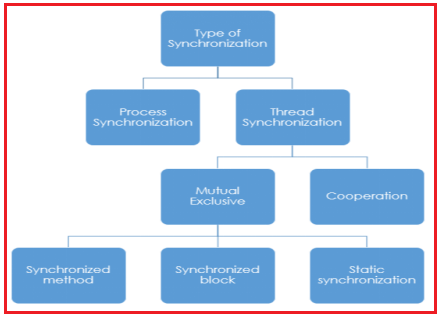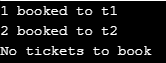## Thread Synchronization in Java with Examples

The process of allowing multiple threads to modify an object in a sequence is called synchronization. We can allow multiple threads to modify the objects sequentially only by executing that objects’ mutator methods logic in sequence from multiple threads. This is possible by using an object locking concept.

Thread Synchronization is a process of allowing only one thread to use the object when multiple threads are trying to use the particular object at the same time. To achieve this Thread Synchronization we have to use a java keyword or modifier called “synchronized”. Synchronization in java is the capability to control the access of multiple threads to any shared resource. Java Synchronization is a better option where we want to allow only one thread to access the shared resource.

General Syntax:
synchronized(objectidentifier)
{
// Access shared variables and other shared resources;
}

##### Why use Synchronization?

The synchronization is mainly used to :

1. If you start with at least two threads inside a program, there might be a chance when multiple threads attempt to get to the same resource.
2. It can even create an unexpected outcome because of concurrency issues.
##### Types of Synchronization

There are basically two types of synchronization available. They are:

1. Process Synchronization: It means sharing system resources by processes in such a way that, Concurrent access to shared data is handled thereby minimizing the chance of inconsistent data.
##### Locks in Java

Synchronization is built around an internal entity known as the lock or monitor lock. (The API specification often refers to this entity simply as a “monitor.”) Locks play a role in both aspects of synchronization: enforcing exclusive access to an object’s state and establishing happens-before relationships that are essential to visibility.

Every object has a lock associated with it. By convention, a thread that needs exclusive and consistent access to an object’s fields has to acquire the object’s lock before accessing them, and then release the lock when it’s done with them. A thread is said to own the lock between the time it has acquired the lock and released the lock. As long as a thread owns a lock, no other thread can acquire the same lock. The other thread will block when it attempts to acquire the lock.

##### Reentrant Synchronization

A thread cannot acquire a lock owned by another thread. But a thread can acquire a lock that it already owns. Allowing a thread to acquire the same lock more than once enables reentrant synchronization. This describes a situation where synchronized code, directly or indirectly, invokes a method that also contains synchronized code, and both sets of code use the same lock. Without reentrant synchronization, synchronized code would have to take many additional precautions to avoid having a thread cause itself to block.

##### Understanding the problem without synchronization

In this example, we are not using synchronization and creating multiple threads that are accessing the display method and producing random output.

```public class Synchronization implements Runnable
{
int tickets = 3;
static int i = 1, j = 2, k = 3;
public void bookticket (String name, int wantedtickets)
{
if (wantedtickets <= tickets)
{
System.out.println (wantedtickets + " booked to " + name);
tickets = tickets - wantedtickets;
}
else
{
System.out.println ("No tickets to book");
}
}
public void run ()
{
if (name.equals ("t1"))
{
bookticket (name, i);
}
else if (name.equals ("t2"))
{
bookticket (name, j);
}
else
{
bookticket (name, k);
}
}
public static void main (String[]args)
{
Synchronization s = new Synchronization ();
t1.setName ("t1");
t2.setName ("t2");
t3.setName ("t3");
t1.start ();
t2.start ();
t3.start ();
}
}```
###### Output:In the above program, object s of class Synchronization are shared by all the three running threads(t1, t2, and t3) to call the shared method(void bookticket). Hence the result is non-synchronized and such a situation is called a Race condition.

##### Synchronized keyword

Java synchronized keyword marks a block or a method in a critical section. A critical section is where only one thread is executing at a time, and the thread holds the lock for the synchronized section. This synchronized keyword helps in writing concurrent parts of any application. It also protects shared resources within the block.

To overcome the above Race Condition problem, we must synchronize access to the shared display() method, making it available to only one thread at a time. This is done by using the keyword synchronized with display() method, i.e. Synchronized void display(String msg)

There are two types of Thread Synchronization. They are:

1. Mutual Exclusive
2. Cooperation (Inter-Thread Communication) (I will discuss in the next article)
##### Mutual Exclusive:

Mutual Exclusive helps keep threads from interfering with one another while sharing data. Mutual Exclusion can be achieved in three ways in java:

1. Synchronized Method
2. Synchronized Block
3. Static Synchronization##### Thread Synchronization using Synchronized Method in Java:

Method level synchronization is used for making a method code thread-safe, i.e. only one thread must be executing this method code.

Syntax :
<access modifier> synchronized method ( parameter)
{
//synchronized code
}

In the case of the synchronized method, the lock object is:

1. class object – if the given method is static.
2. this object – if the method is non-static. ‘this’ is the reference to the current object in which the synchronized method is invoked.
##### Example: Implementation of Synchronized Method in Java
```public class Synchronization implements Runnable
{
int tickets = 3;
static int i = 1, j = 2, k = 3;
synchronized void bookticket (String name, int wantedtickets)
{
if (wantedtickets <= tickets)
{
System.out.println (wantedtickets + " booked to " + name);
tickets = tickets - wantedtickets;
}
else
{
System.out.println ("No tickets to book");
}
}
public void run ()
{
if (name.equals ("t1"))
{
bookticket (name, i);
}
else if (name.equals ("t2"))
{
bookticket (name, j);
}
else
{
bookticket (name, k);
}
}

public static void main (String[]args)
{
Synchronization s = new Synchronization ();
t1.setName ("t1");
t2.setName ("t2");
t3.setName ("t3");
t1.start ();
t2.start ();
t3.start ();
}
}```
###### Output:##### Thread Synchronization using Synchronized Block in Java:

Block-level synchronization is used for making some amount of method code thread-safe. If we want to synchronize access to an object of a class or only a part of a method to be synchronized then we can use the synchronized block for it. It is capable to make any part of the object and method synchronized.

Syntax:
synchronized (object reference expression) {
//code block
}

##### Example: Implementation of Synchronized Block in java
```class A implements Runnable
{
int token = 1;
public void run ()
{
synchronized (this)
{
String name = t.getName ();
System.out.println (token + ".....alloted to " + name);
token++;
}
}
}
class SynchroBlock
{
public static void main (String[]args)
{
A a1 = new A ();
t1.setName ("t1");
t2.setName ("t2");
t3.setName ("t3");
t1.start ();
t2.start ();
t3.start ();
}
}```
###### Output:##### Thread Synchronization using Static Synchronized in Java:

In simple words, a static synchronized method will lock the class instead of the object, and it will lock the class because the keyword static means: “class instead of instance”. The keyword synchronized means that only one thread can access the method at a time. And static synchronized mean: Only one thread can access the class at one time.

##### Example: Implementation of Static Synchronized in Java
```class Table
{
synchronized static void printTable (int n)
{
for (int i = 1; i <= 10; i++)
{
System.out.println (n * i);
try
{
}
catch (Exception e)
{
}
}
}
}

{
public void run ()
{
Table.printTable (1);
}
}

{
public void run ()
{
Table.printTable (10);
}
}

{
public void run ()
{
Table.printTable (100);
}
}

{
public void run ()
{
Table.printTable (1000);
}
}

public class StaticSynchronization
{
public static void main (String t[])
{
t1.start ();
t2.start ();
t3.start ();
t4.start ();
}
}```

Thread joining mechanism is applied only for the threads which are created statically (i.e., dependent on one another) and whereas synchronization is for thread locking dynamically.

##### What happens when we declare Methods are synchronized?

When we call the synchronized method the current object of this method is locked by this current thread. So that other threads cannot use this locked object for executing either the same synchronized method or other synchronized methods. But it is possible to access non-synchronized methods by using the locked object because to execute a non-synchronized methods object is no need to be locked by this thread. The threads that lock the object is called monitor. This current object is unlocked only after completion of that synchronized method execution either normally or abnormally.

##### What is the difference between synchronized blocks and Methods in java?

If a method is declared as synchronized that method’s complete logic is executed in sequence from multiple threads by using the same object. If we declare the block as synchronized, only the statements written inside that block are executed sequentially not complete method logic.

Using a synchronized method we can only lock the current object of the method. Using a synchronized block we can lock either the current object or the argument object of the method. We must pass the object’s reference variable to the synchronized block as shown below.

###### Locking the current object:

class Example
{
void m1()
{
synchronized (this)
{}
}
}

###### Locking argument object:

class Example
{
void m1(sample s)
{
synchronized (s)
{}
}
}

Note: We can develop any number of synchronized blocks in a method.

###### When should we develop multiple synchronized blocks instead of declaring the complete method as synchronized?

In the below two cases we develop multiple synchronized blocks

1. For executing parts of method logic sequentially for doing object modification
2. For executing one part of method logic by locking the current object and other parts of the method by locking the argument object.
###### What is the Difference between Declaring Non-Static Method and Static Method as synchronized?

If we declare the Non-Static Method as synchronized its current object is locked. So that in this method Non-Static Variables s of this object are modified sequentially by multiple threads. If we declare SM as synchronized its class’s java.lang. A class object is locked so that in this method Static Variables are modified sequentially by multiple threads.

In the next article, I am going to discuss Inter Thread Communication in Java with Examples. Here, in this article, I try to explain Thread Synchronization in Java with Examples. I hope you enjoy this Thread Synchronization in Java with Examples article.